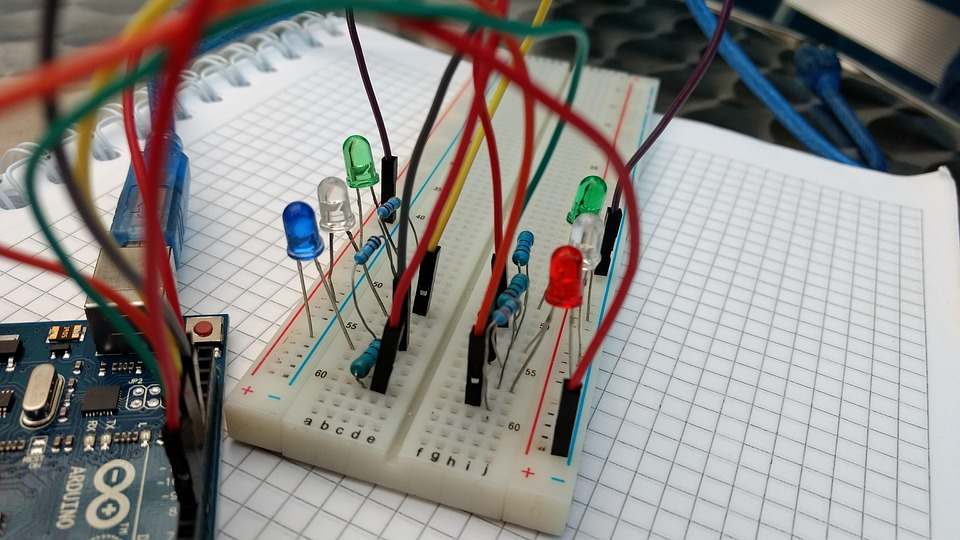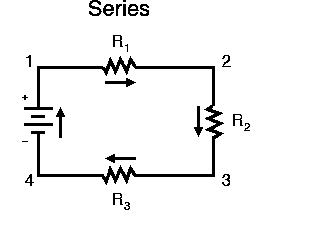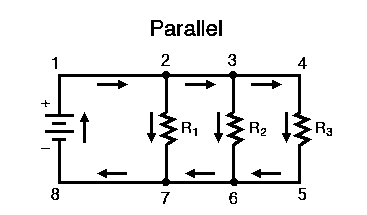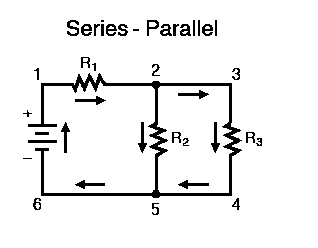# What is series and parallel circuitSeries Circuit:

A series circuit is a configuration in which multiple components (such as resistors, bulbs, or other devices) are connected end-to-end in a single path for the electric current to flow. In a series circuit, the same current passes through all the components. If one component fails (open circuit), the entire circuit is interrupted, and the current stops flowing. Below is an example of series circuit:Here, we have three resistors R1, R2, and R3 are connected in a string from one terminal of the battery to the other wherein the current flows in a clockwise direction through a single path, from point 1 to point 2 to point 3 to point 4 and back around to 1.

Parallel Circuit:

A parallel circuit is a configuration in which multiple components are connected across the same two points, creating multiple paths for the electric current to flow. In a parallel circuit, the voltage across all components is the same, but the current is divided among the branches. If one component fails, the other branches are unaffected, and the circuit remains closed. Below is an example of a Parallel Circuit:In this circuit, resistors R1, R2 and R3 are forming more than one continuous path to flow current. There’s one path from 1 to 2 to 7 to 8 and back to 1 again. There’s another path from 1 to 2 to 3 to 6 to 7 to 8 and back to 1 again. And then there’s a third path from 1 to 2 to 3 to 4 to 5 to 6 to 7 to 8 and back to 1 again. Each individual path (through R1, R2, and R3) is called a branch. Here, all components are connected between the same set of electrically common points or nodes. Point or node 1, 2, 3, and 4 are all electrically common. Same thing is applicable for the point or node 8, 7, 6, and 5. In this circuit, all resistors and the battery are connected between these two sets of points or nodes.

Example of a Series-Parallel Configuration CircuitThere are two loops for the current to flow through in this circuit. One loop is from point or node 1 to 2 to 5 to 6 and back to 1 again, and another loop is from the point or node 1 to 2 to 3 to 4 to 5 to 6 and back to 1 again. Notice how both current paths pass through R1 (from point or node 1 to point or node 2). In this circuit, resistors R2 and R3 are in parallel with each other having another resistor R1 in series with the parallel combination of R2 and R3.

In series circuit, current remains same, but voltage drops. In parallel circuit, voltage remains same, but current is divided in many paths.

Comparison:

• Voltage: In a series circuit, the voltage is divided among the components. In a parallel circuit, all components share the same voltage.

• Current: In a series circuit, the same current flows through all components. In a parallel circuit, the total current is divided among the branches.

• Resistance: In a series circuit, the total resistance is the sum of individual resistances. In a parallel circuit, the reciprocal of the total resistance is the sum of the reciprocals of individual resistances.

• Brightness: In a series circuit with bulbs, adding more bulbs increases resistance, leading to dimmer bulbs. In a parallel circuit with bulbs, adding more bulbs does not affect the brightness of the others.

• Reliability: Series circuits are less reliable as a single component failure interrupts the entire circuit. Parallel circuits are more reliable as one branch can fail without affecting others.

• Applications: Series circuits are used in simple applications like string lights. Parallel circuits are used in most household electrical systems and electronics.

Remember, when designing electrical circuits, the choice between series and parallel connections depends on the specific requirements of the circuit and the intended functionality of the components.

Watch Video: What is Series and Parallel Circuit?

Prasun Barua

Prasun Barua is an Engineer (Electrical & Electronic) and Member of the European Energy Centre (EEC). His first published book Green Planet is all about green technologies and science. His other published books are Solar PV System Design and Technology, Electricity from Renewable Energy, Tech Know Solar PV System, C Coding Practice, AI and Robotics Overview, Robotics and Artificial Intelligence, Know How Solar PV System, Know The Product, Solar PV Technology Overview, Home Appliances Overview, Tech Know Solar PV System, C Programming Practice, etc. These books are available at Google Books, Google Play, Amazon and other platforms.

*